Скачать презентацию 11 1 The Simple Interest Formula n Find

6b882d46e2cf612216b25522df9b43e4.ppt

• Количество слайдов: 45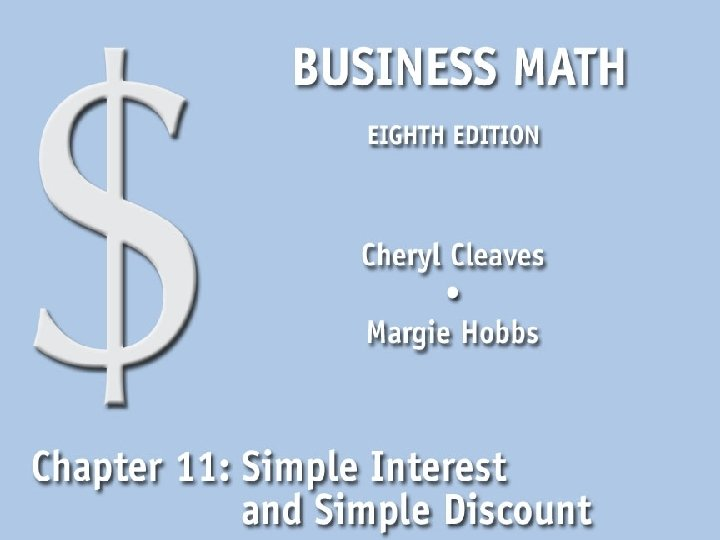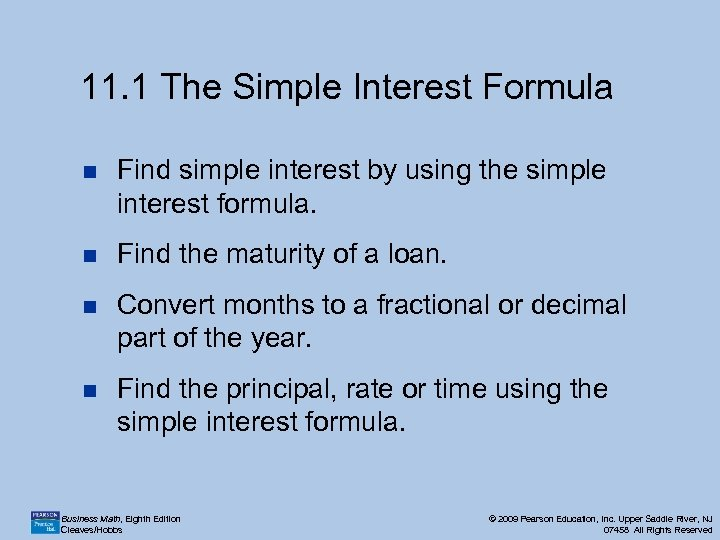11. 1 The Simple Interest Formula n Find simple interest by using the simple interest formula. n Find the maturity of a loan. n Convert months to a fractional or decimal part of the year. n Find the principal, rate or time using the simple interest formula. Business Math, Eighth Edition Cleaves/Hobbs © 2009 Pearson Education, Inc. Upper Saddle River, NJ 07458 All Rights Reserved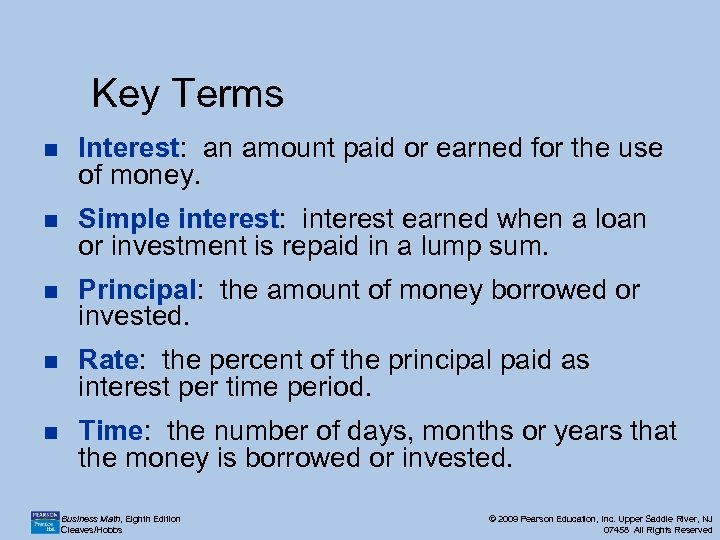Key Terms n Interest: an amount paid or earned for the use of money. n Simple interest: interest earned when a loan or investment is repaid in a lump sum. n Principal: the amount of money borrowed or invested. n Rate: the percent of the principal paid as interest per time period. n Time: the number of days, months or years that the money is borrowed or invested. Business Math, Eighth Edition Cleaves/Hobbs © 2009 Pearson Education, Inc. Upper Saddle River, NJ 07458 All Rights Reserved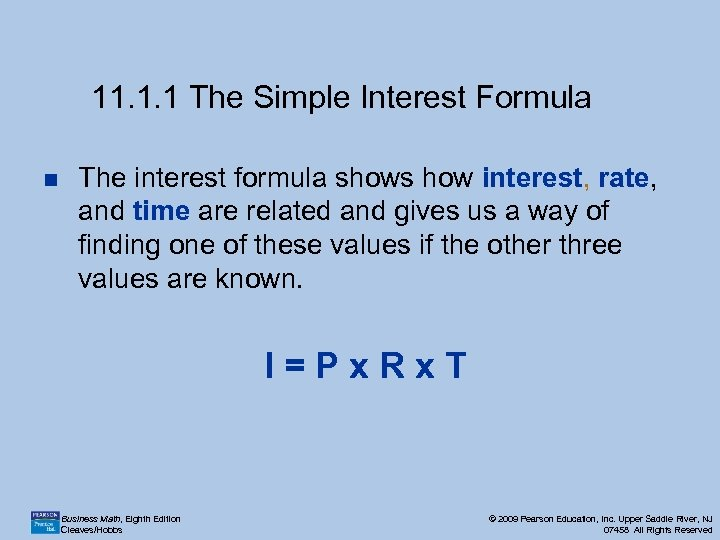11. 1. 1 The Simple Interest Formula n The interest formula shows how interest, rate, and time are related and gives us a way of finding one of these values if the other three values are known. I=Px. Rx. T Business Math, Eighth Edition Cleaves/Hobbs © 2009 Pearson Education, Inc. Upper Saddle River, NJ 07458 All Rights Reserved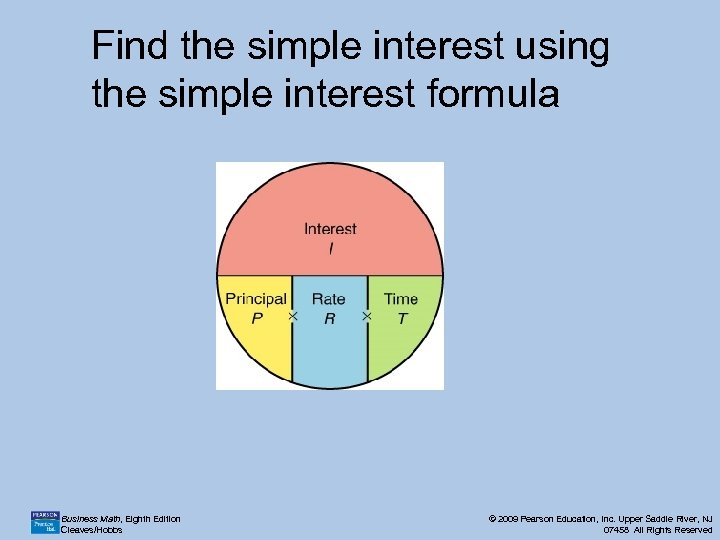Find the simple interest using the simple interest formula Business Math, Eighth Edition Cleaves/Hobbs © 2009 Pearson Education, Inc. Upper Saddle River, NJ 07458 All Rights Reserved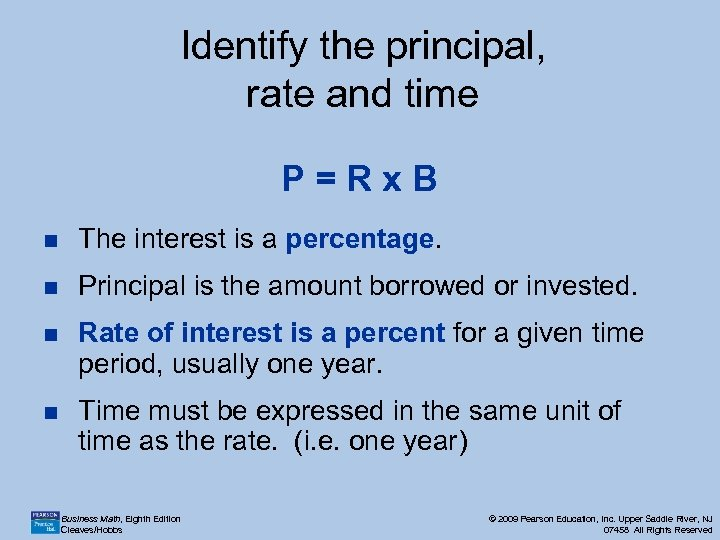Identify the principal, rate and time P=Rx. B n The interest is a percentage. n Principal is the amount borrowed or invested. n Rate of interest is a percent for a given time period, usually one year. n Time must be expressed in the same unit of time as the rate. (i. e. one year) Business Math, Eighth Edition Cleaves/Hobbs © 2009 Pearson Education, Inc. Upper Saddle River, NJ 07458 All Rights Reserved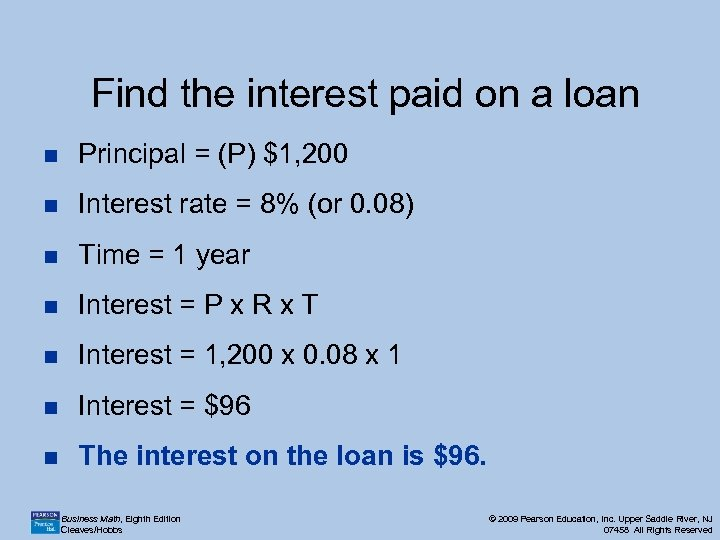Find the interest paid on a loan n Principal = (P) \$1, 200 n Interest rate = 8% (or 0. 08) n Time = 1 year n Interest = P x R x T n Interest = 1, 200 x 0. 08 x 1 n Interest = \$96 n The interest on the loan is \$96. Business Math, Eighth Edition Cleaves/Hobbs © 2009 Pearson Education, Inc. Upper Saddle River, NJ 07458 All Rights Reserved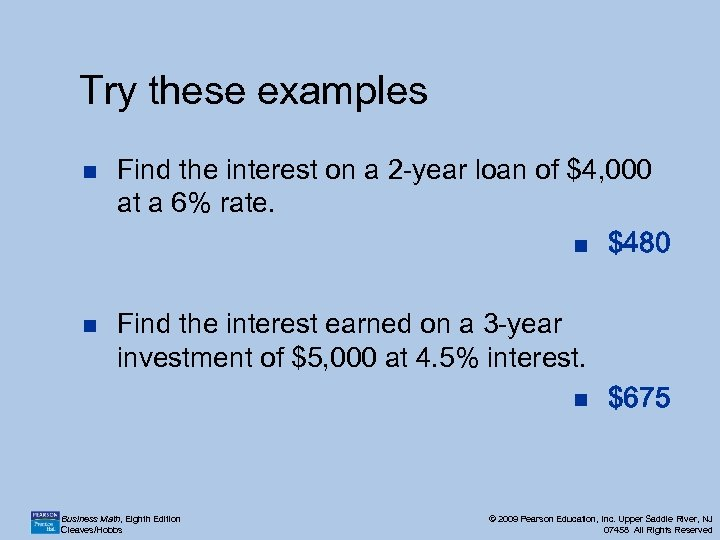Try these examples n n Find the interest on a 2 -year loan of \$4, 000 at a 6% rate. n \$480 Find the interest earned on a 3 -year investment of \$5, 000 at 4. 5% interest. n Business Math, Eighth Edition Cleaves/Hobbs \$675 © 2009 Pearson Education, Inc. Upper Saddle River, NJ 07458 All Rights Reserved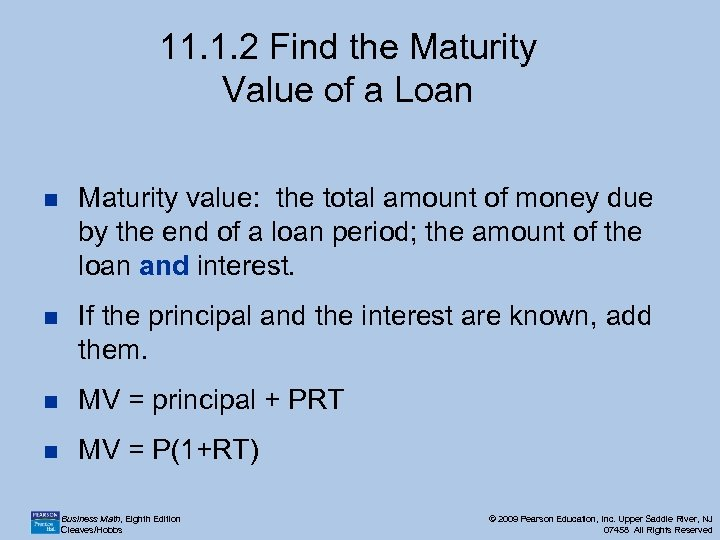11. 1. 2 Find the Maturity Value of a Loan n Maturity value: the total amount of money due by the end of a loan period; the amount of the loan and interest. n If the principal and the interest are known, add them. n MV = principal + PRT n MV = P(1+RT) Business Math, Eighth Edition Cleaves/Hobbs © 2009 Pearson Education, Inc. Upper Saddle River, NJ 07458 All Rights Reserved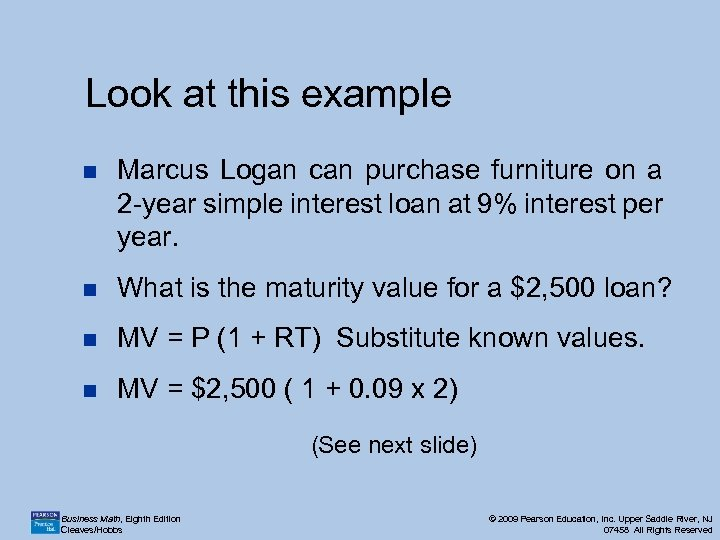Look at this example n Marcus Logan can purchase furniture on a 2 -year simple interest loan at 9% interest per year. n What is the maturity value for a \$2, 500 loan? n MV = P (1 + RT) Substitute known values. n MV = \$2, 500 ( 1 + 0. 09 x 2) (See next slide) Business Math, Eighth Edition Cleaves/Hobbs © 2009 Pearson Education, Inc. Upper Saddle River, NJ 07458 All Rights Reserved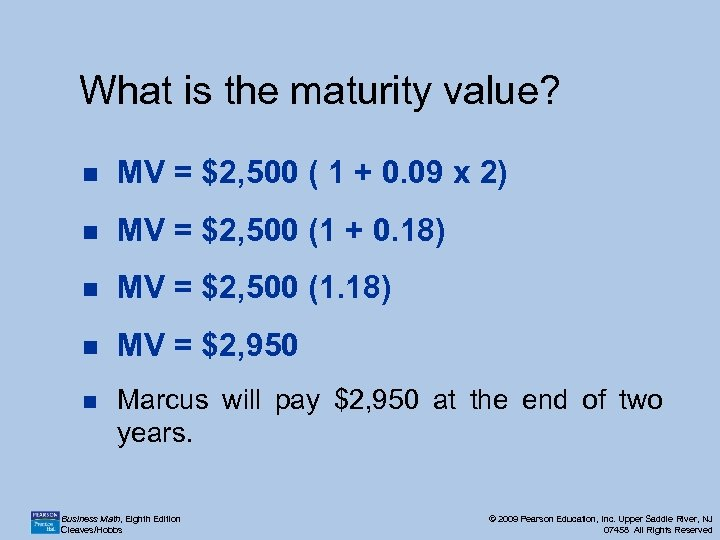What is the maturity value? n MV = \$2, 500 ( 1 + 0. 09 x 2) n MV = \$2, 500 (1 + 0. 18) n MV = \$2, 500 (1. 18) n MV = \$2, 950 n Marcus will pay \$2, 950 at the end of two years. Business Math, Eighth Edition Cleaves/Hobbs © 2009 Pearson Education, Inc. Upper Saddle River, NJ 07458 All Rights Reserved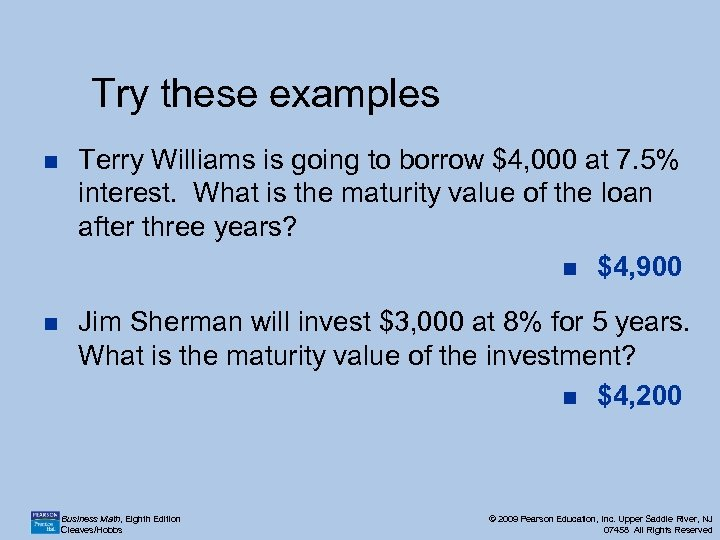Try these examples n Terry Williams is going to borrow \$4, 000 at 7. 5% interest. What is the maturity value of the loan after three years? n \$4, 900 n Jim Sherman will invest \$3, 000 at 8% for 5 years. What is the maturity value of the investment? n \$4, 200 Business Math, Eighth Edition Cleaves/Hobbs © 2009 Pearson Education, Inc. Upper Saddle River, NJ 07458 All Rights Reserved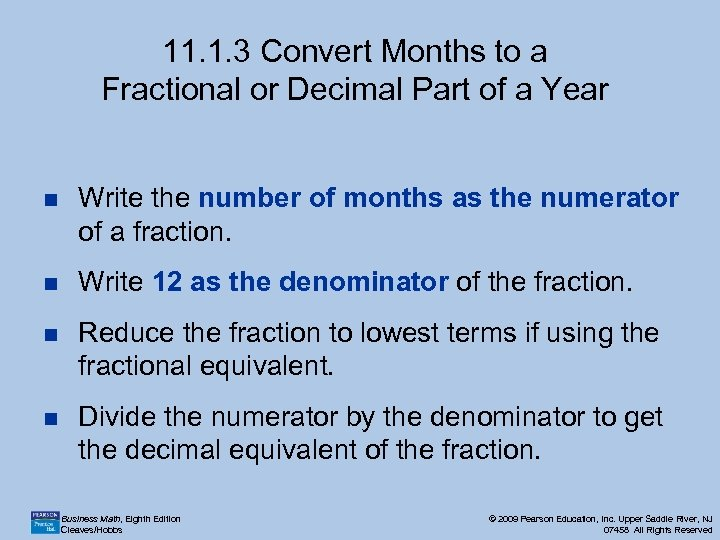11. 1. 3 Convert Months to a Fractional or Decimal Part of a Year n Write the number of months as the numerator of a fraction. n Write 12 as the denominator of the fraction. n Reduce the fraction to lowest terms if using the fractional equivalent. n Divide the numerator by the denominator to get the decimal equivalent of the fraction. Business Math, Eighth Edition Cleaves/Hobbs © 2009 Pearson Education, Inc. Upper Saddle River, NJ 07458 All Rights Reserved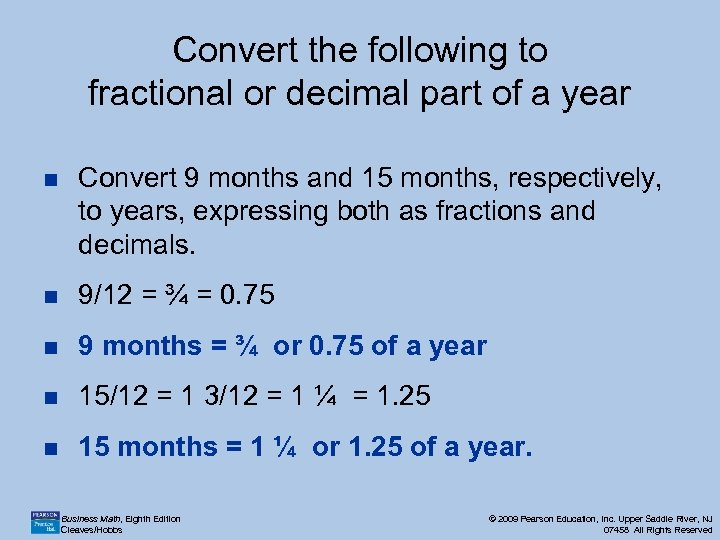Convert the following to fractional or decimal part of a year n Convert 9 months and 15 months, respectively, to years, expressing both as fractions and decimals. n 9/12 = ¾ = 0. 75 n 9 months = ¾ or 0. 75 of a year n 15/12 = 1 3/12 = 1 ¼ = 1. 25 n 15 months = 1 ¼ or 1. 25 of a year. Business Math, Eighth Edition Cleaves/Hobbs © 2009 Pearson Education, Inc. Upper Saddle River, NJ 07458 All Rights Reserved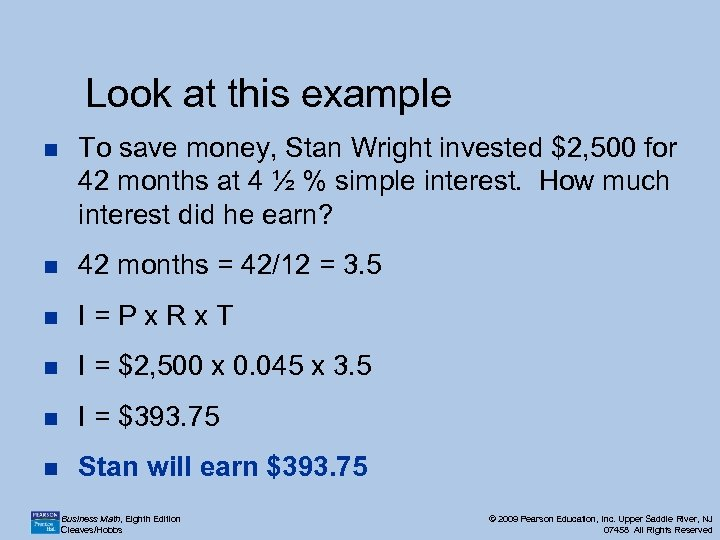Look at this example n To save money, Stan Wright invested \$2, 500 for 42 months at 4 ½ % simple interest. How much interest did he earn? n 42 months = 42/12 = 3. 5 n I=Px. Rx. T n I = \$2, 500 x 0. 045 x 3. 5 n I = \$393. 75 n Stan will earn \$393. 75 Business Math, Eighth Edition Cleaves/Hobbs © 2009 Pearson Education, Inc. Upper Saddle River, NJ 07458 All Rights Reserved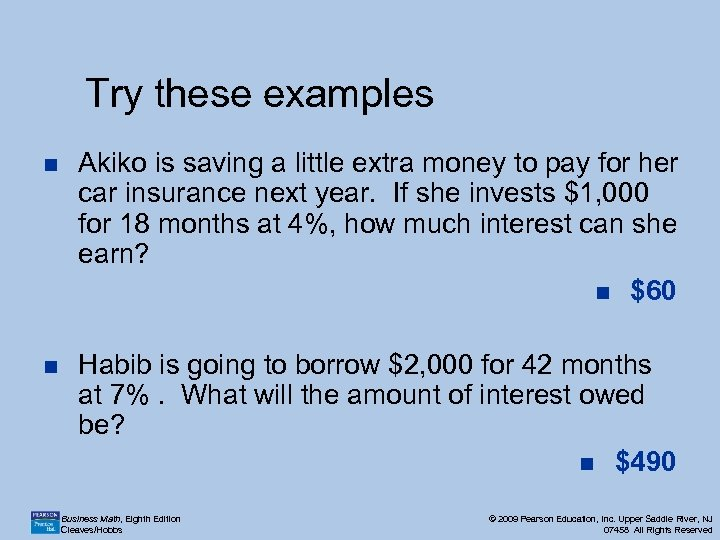Try these examples n Akiko is saving a little extra money to pay for her car insurance next year. If she invests \$1, 000 for 18 months at 4%, how much interest can she earn? n \$60 n Habib is going to borrow \$2, 000 for 42 months at 7%. What will the amount of interest owed be? n \$490 Business Math, Eighth Edition Cleaves/Hobbs © 2009 Pearson Education, Inc. Upper Saddle River, NJ 07458 All Rights Reserved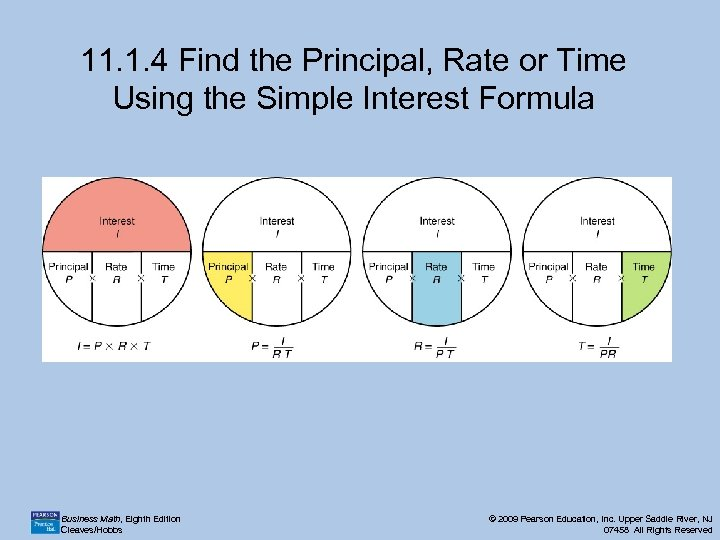11. 1. 4 Find the Principal, Rate or Time Using the Simple Interest Formula Business Math, Eighth Edition Cleaves/Hobbs © 2009 Pearson Education, Inc. Upper Saddle River, NJ 07458 All Rights Reserved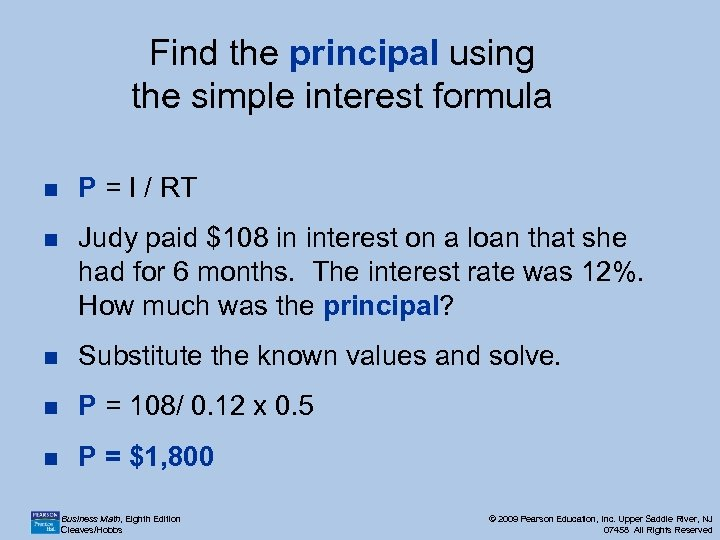Find the principal using the simple interest formula n P = I / RT n Judy paid \$108 in interest on a loan that she had for 6 months. The interest rate was 12%. How much was the principal? n Substitute the known values and solve. n P = 108/ 0. 12 x 0. 5 n P = \$1, 800 Business Math, Eighth Edition Cleaves/Hobbs © 2009 Pearson Education, Inc. Upper Saddle River, NJ 07458 All Rights Reserved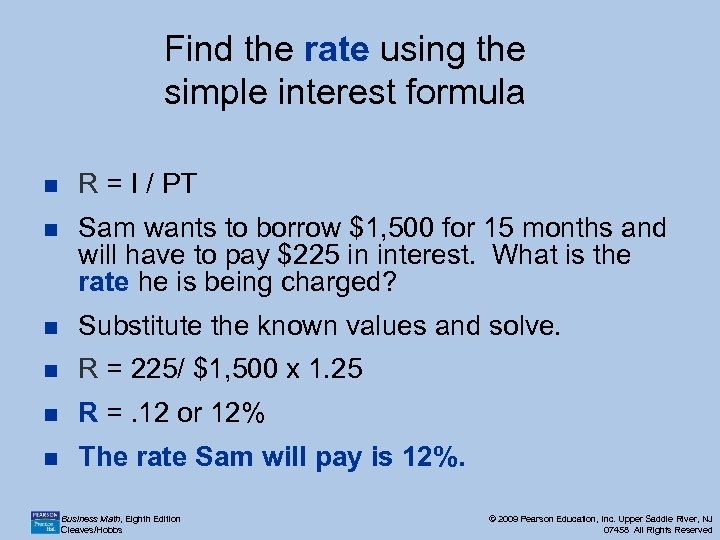Find the rate using the simple interest formula n R = I / PT n Sam wants to borrow \$1, 500 for 15 months and will have to pay \$225 in interest. What is the rate he is being charged? n Substitute the known values and solve. n R = 225/ \$1, 500 x 1. 25 n R =. 12 or 12% n The rate Sam will pay is 12%. Business Math, Eighth Edition Cleaves/Hobbs © 2009 Pearson Education, Inc. Upper Saddle River, NJ 07458 All Rights Reserved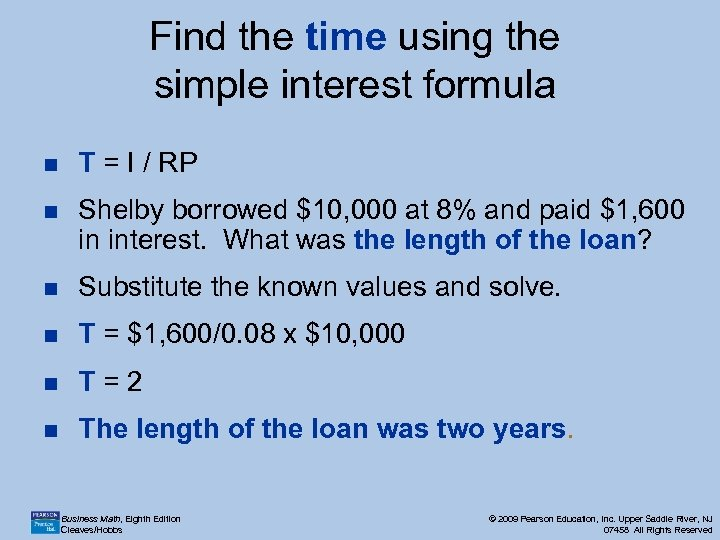Find the time using the simple interest formula n T = I / RP n Shelby borrowed \$10, 000 at 8% and paid \$1, 600 in interest. What was the length of the loan? n Substitute the known values and solve. n T = \$1, 600/0. 08 x \$10, 000 n T=2 n The length of the loan was two years. Business Math, Eighth Edition Cleaves/Hobbs © 2009 Pearson Education, Inc. Upper Saddle River, NJ 07458 All Rights Reserved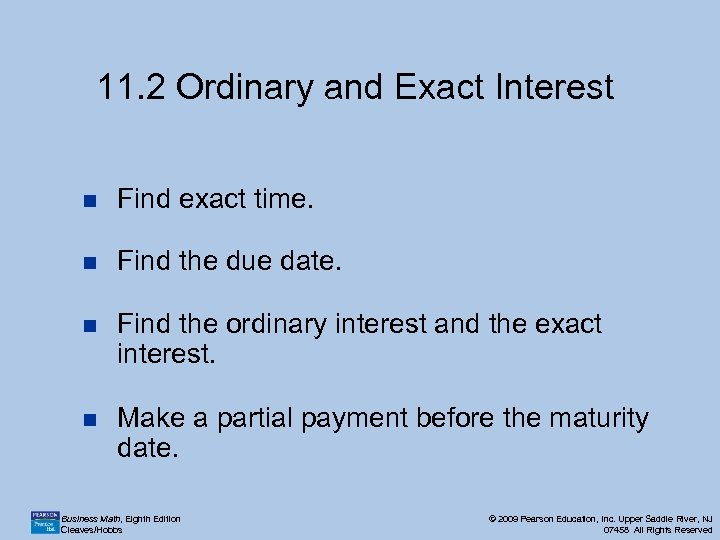11. 2 Ordinary and Exact Interest n Find exact time. n Find the due date. n Find the ordinary interest and the exact interest. n Make a partial payment before the maturity date. Business Math, Eighth Edition Cleaves/Hobbs © 2009 Pearson Education, Inc. Upper Saddle River, NJ 07458 All Rights Reserved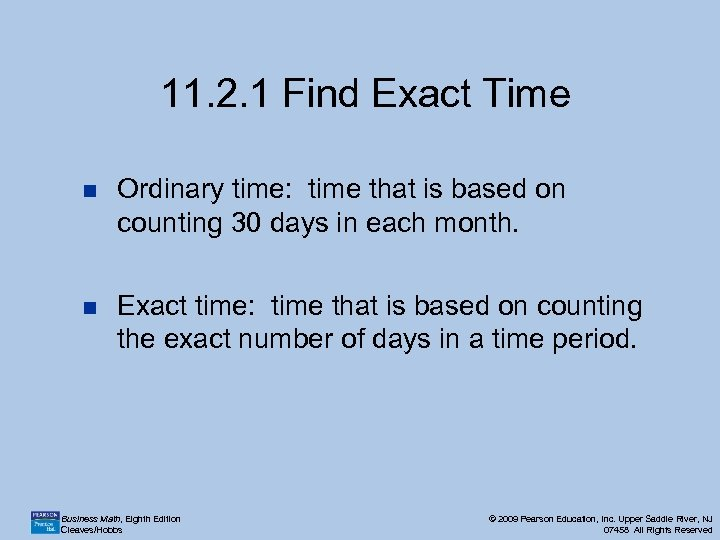11. 2. 1 Find Exact Time n Ordinary time: time that is based on counting 30 days in each month. n Exact time: time that is based on counting the exact number of days in a time period. Business Math, Eighth Edition Cleaves/Hobbs © 2009 Pearson Education, Inc. Upper Saddle River, NJ 07458 All Rights Reserved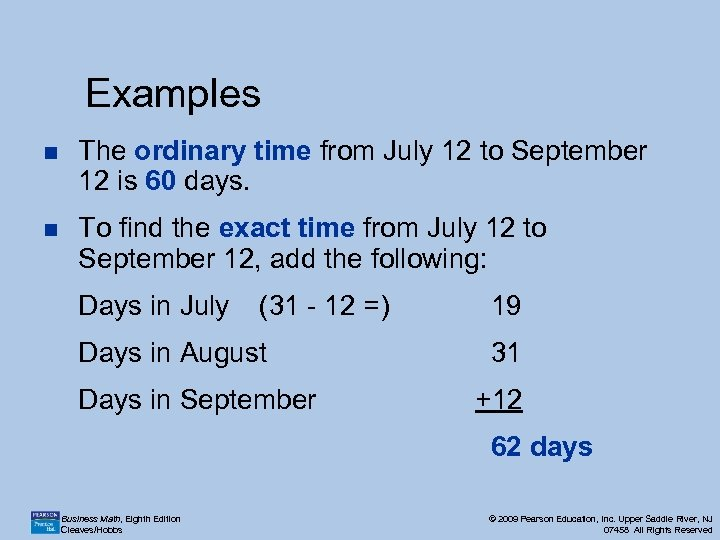Examples n The ordinary time from July 12 to September 12 is 60 days. n To find the exact time from July 12 to September 12, add the following: Days in July (31 - 12 =) Days in August Days in September 19 31 +12 62 days Business Math, Eighth Edition Cleaves/Hobbs © 2009 Pearson Education, Inc. Upper Saddle River, NJ 07458 All Rights Reserved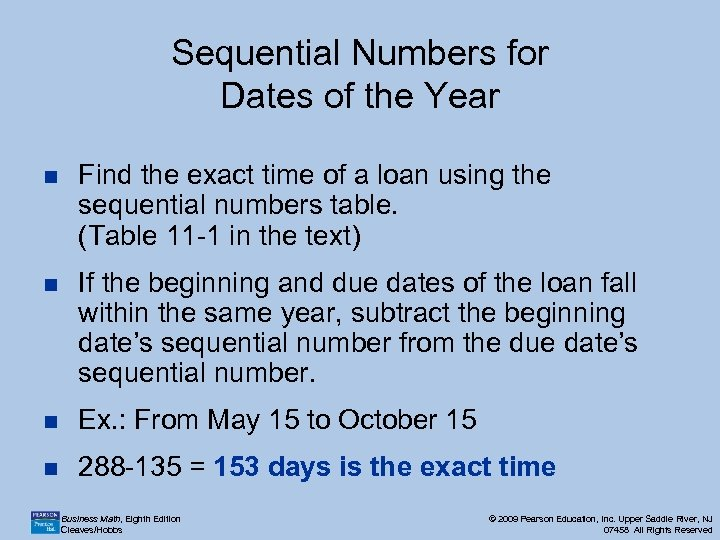Sequential Numbers for Dates of the Year n Find the exact time of a loan using the sequential numbers table. (Table 11 -1 in the text) n If the beginning and due dates of the loan fall within the same year, subtract the beginning date’s sequential number from the due date’s sequential number. n Ex. : From May 15 to October 15 n 288 -135 = 153 days is the exact time Business Math, Eighth Edition Cleaves/Hobbs © 2009 Pearson Education, Inc. Upper Saddle River, NJ 07458 All Rights Reserved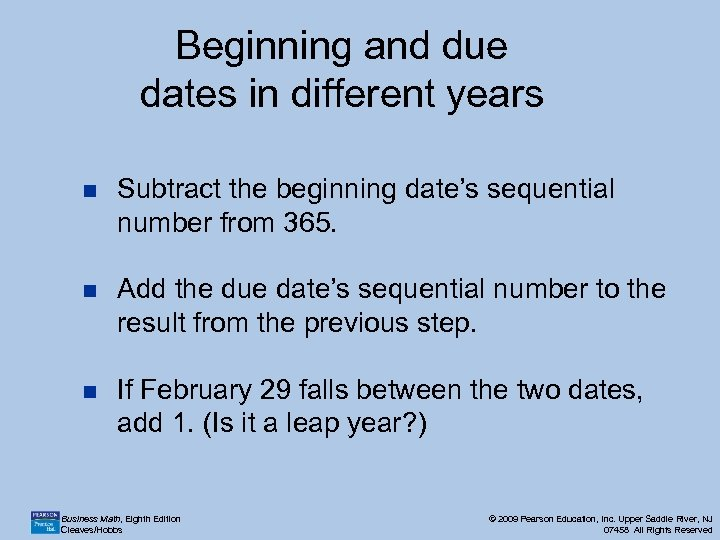Beginning and due dates in different years n Subtract the beginning date’s sequential number from 365. n Add the due date’s sequential number to the result from the previous step. n If February 29 falls between the two dates, add 1. (Is it a leap year? ) Business Math, Eighth Edition Cleaves/Hobbs © 2009 Pearson Education, Inc. Upper Saddle River, NJ 07458 All Rights Reserved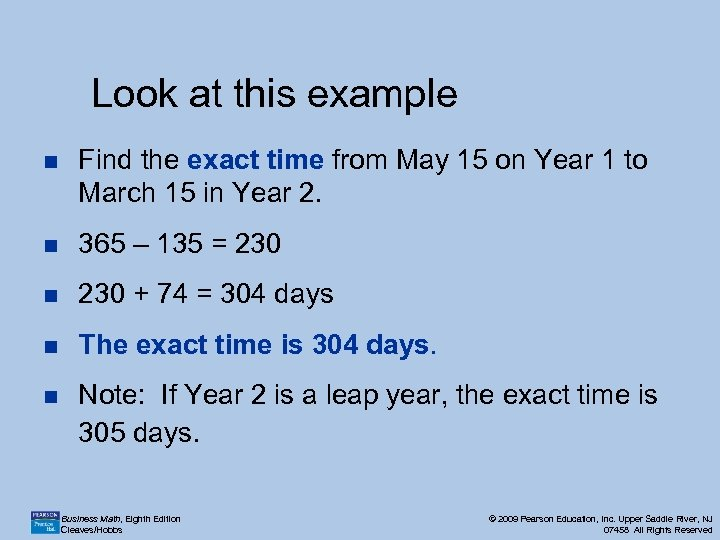Look at this example n Find the exact time from May 15 on Year 1 to March 15 in Year 2. n 365 – 135 = 230 n 230 + 74 = 304 days n The exact time is 304 days. n Note: If Year 2 is a leap year, the exact time is 305 days. Business Math, Eighth Edition Cleaves/Hobbs © 2009 Pearson Education, Inc. Upper Saddle River, NJ 07458 All Rights Reserved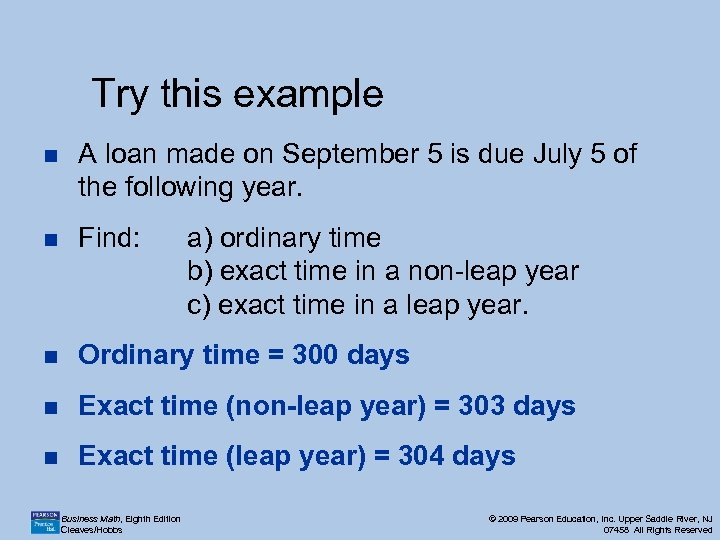Try this example n A loan made on September 5 is due July 5 of the following year. n Find: n Ordinary time = 300 days n Exact time (non-leap year) = 303 days n Exact time (leap year) = 304 days Business Math, Eighth Edition Cleaves/Hobbs a) ordinary time b) exact time in a non-leap year c) exact time in a leap year. © 2009 Pearson Education, Inc. Upper Saddle River, NJ 07458 All Rights Reserved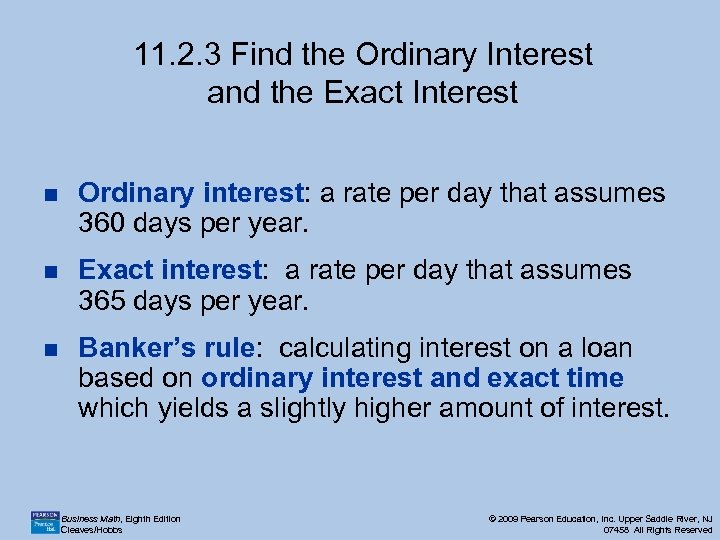11. 2. 3 Find the Ordinary Interest and the Exact Interest n Ordinary interest: a rate per day that assumes 360 days per year. n Exact interest: a rate per day that assumes 365 days per year. n Banker’s rule: calculating interest on a loan based on ordinary interest and exact time which yields a slightly higher amount of interest. Business Math, Eighth Edition Cleaves/Hobbs © 2009 Pearson Education, Inc. Upper Saddle River, NJ 07458 All Rights Reserved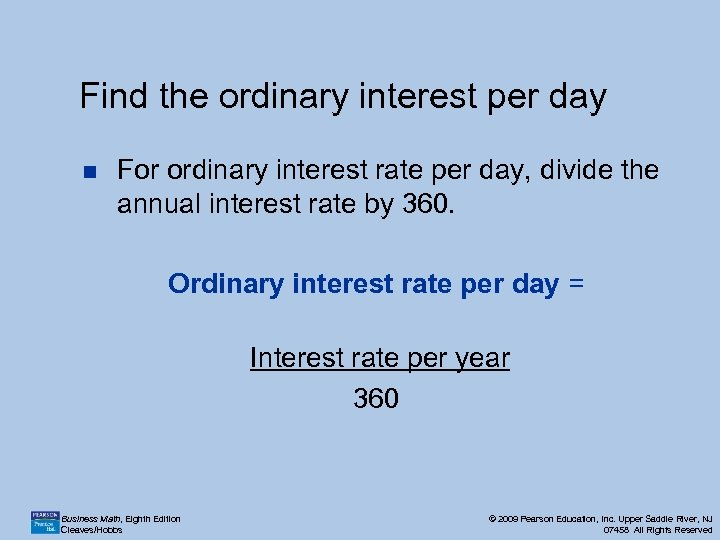Find the ordinary interest per day n For ordinary interest rate per day, divide the annual interest rate by 360. Ordinary interest rate per day = Interest rate per year 360 Business Math, Eighth Edition Cleaves/Hobbs © 2009 Pearson Education, Inc. Upper Saddle River, NJ 07458 All Rights Reserved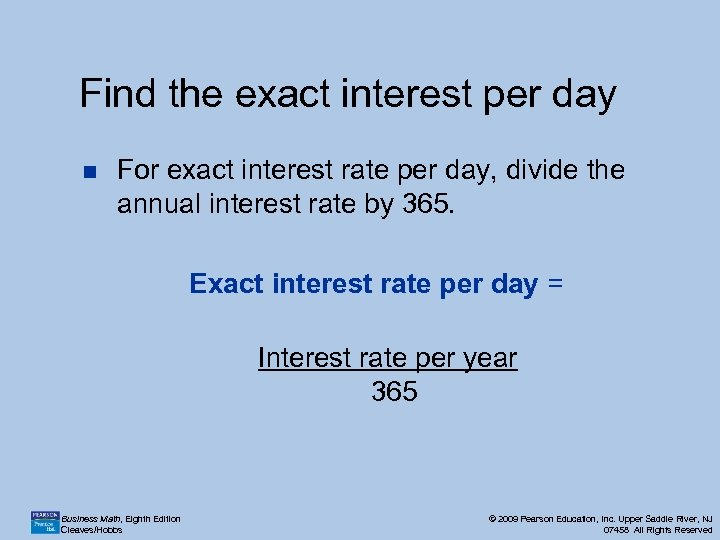Find the exact interest per day n For exact interest rate per day, divide the annual interest rate by 365. Exact interest rate per day = Interest rate per year 365 Business Math, Eighth Edition Cleaves/Hobbs © 2009 Pearson Education, Inc. Upper Saddle River, NJ 07458 All Rights Reserved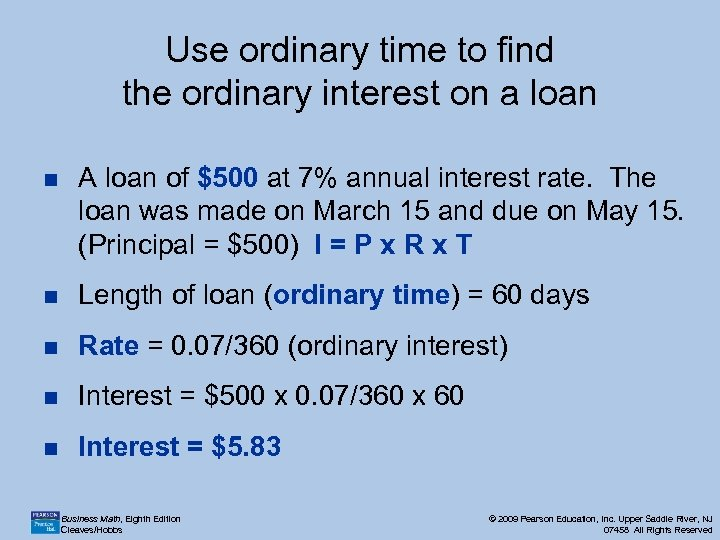Use ordinary time to find the ordinary interest on a loan n A loan of \$500 at 7% annual interest rate. The loan was made on March 15 and due on May 15. (Principal = \$500) I = P x R x T n Length of loan (ordinary time) = 60 days n Rate = 0. 07/360 (ordinary interest) n Interest = \$500 x 0. 07/360 x 60 n Interest = \$5. 83 Business Math, Eighth Edition Cleaves/Hobbs © 2009 Pearson Education, Inc. Upper Saddle River, NJ 07458 All Rights Reserved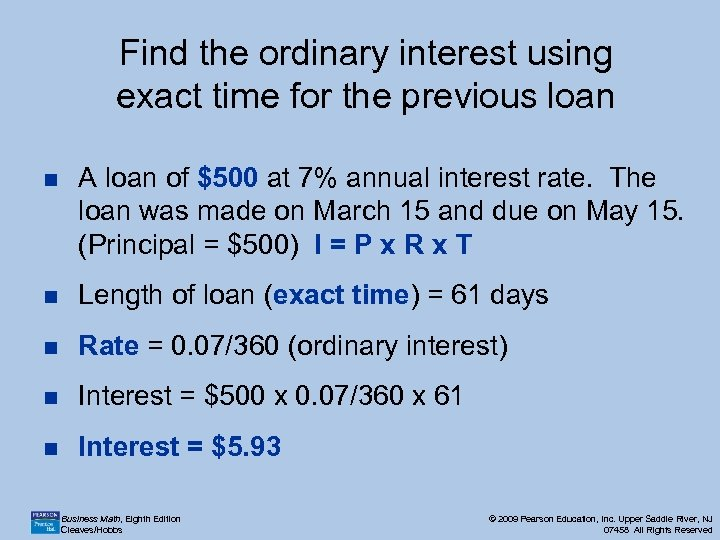Find the ordinary interest using exact time for the previous loan n A loan of \$500 at 7% annual interest rate. The loan was made on March 15 and due on May 15. (Principal = \$500) I = P x R x T n Length of loan (exact time) = 61 days n Rate = 0. 07/360 (ordinary interest) n Interest = \$500 x 0. 07/360 x 61 n Interest = \$5. 93 Business Math, Eighth Edition Cleaves/Hobbs © 2009 Pearson Education, Inc. Upper Saddle River, NJ 07458 All Rights Reserved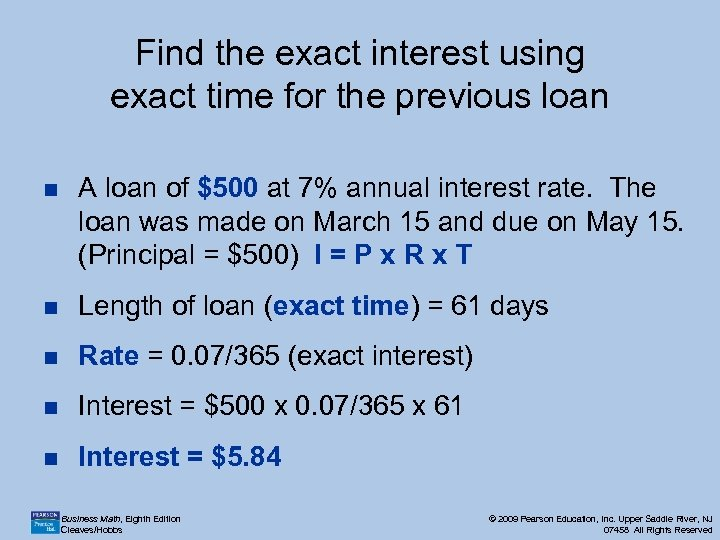Find the exact interest using exact time for the previous loan n A loan of \$500 at 7% annual interest rate. The loan was made on March 15 and due on May 15. (Principal = \$500) I = P x R x T n Length of loan (exact time) = 61 days n Rate = 0. 07/365 (exact interest) n Interest = \$500 x 0. 07/365 x 61 n Interest = \$5. 84 Business Math, Eighth Edition Cleaves/Hobbs © 2009 Pearson Education, Inc. Upper Saddle River, NJ 07458 All Rights Reserved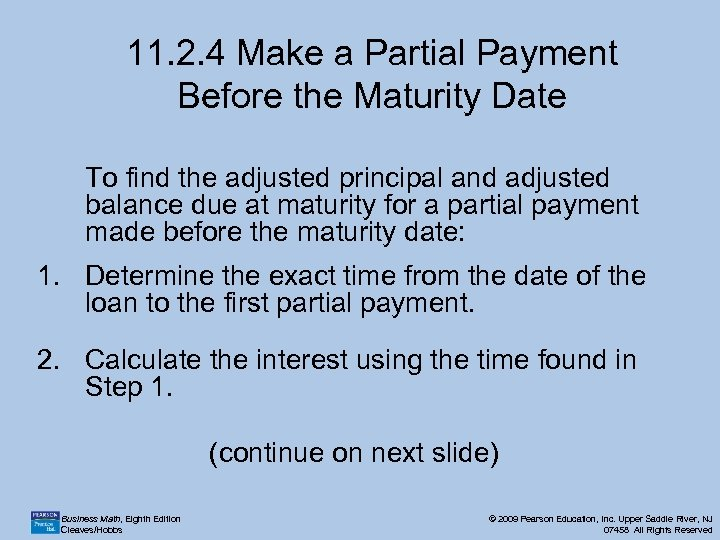11. 2. 4 Make a Partial Payment Before the Maturity Date To find the adjusted principal and adjusted balance due at maturity for a partial payment made before the maturity date: 1. Determine the exact time from the date of the loan to the first partial payment. 2. Calculate the interest using the time found in Step 1. (continue on next slide) Business Math, Eighth Edition Cleaves/Hobbs © 2009 Pearson Education, Inc. Upper Saddle River, NJ 07458 All Rights Reserved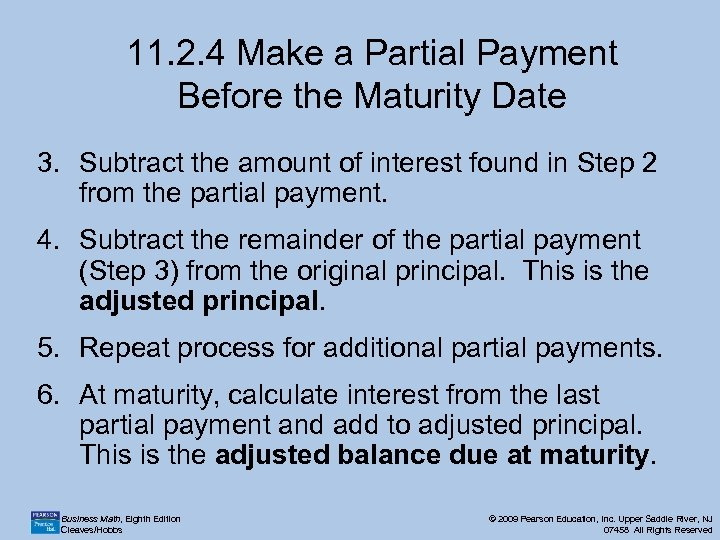11. 2. 4 Make a Partial Payment Before the Maturity Date 3. Subtract the amount of interest found in Step 2 from the partial payment. 4. Subtract the remainder of the partial payment (Step 3) from the original principal. This is the adjusted principal. 5. Repeat process for additional partial payments. 6. At maturity, calculate interest from the last partial payment and add to adjusted principal. This is the adjusted balance due at maturity. Business Math, Eighth Edition Cleaves/Hobbs © 2009 Pearson Education, Inc. Upper Saddle River, NJ 07458 All Rights Reserved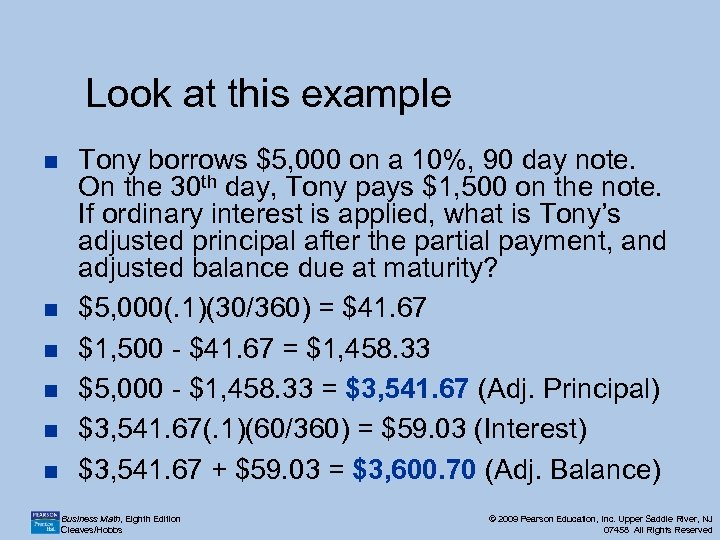Look at this example n n n Tony borrows \$5, 000 on a 10%, 90 day note. On the 30 th day, Tony pays \$1, 500 on the note. If ordinary interest is applied, what is Tony’s adjusted principal after the partial payment, and adjusted balance due at maturity? \$5, 000(. 1)(30/360) = \$41. 67 \$1, 500 - \$41. 67 = \$1, 458. 33 \$5, 000 - \$1, 458. 33 = \$3, 541. 67 (Adj. Principal) \$3, 541. 67(. 1)(60/360) = \$59. 03 (Interest) \$3, 541. 67 + \$59. 03 = \$3, 600. 70 (Adj. Balance) Business Math, Eighth Edition Cleaves/Hobbs © 2009 Pearson Education, Inc. Upper Saddle River, NJ 07458 All Rights Reserved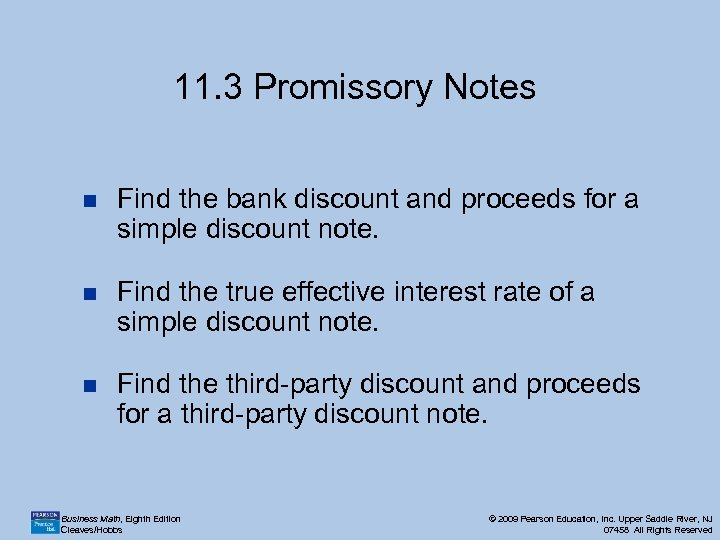11. 3 Promissory Notes n Find the bank discount and proceeds for a simple discount note. n Find the true effective interest rate of a simple discount note. n Find the third-party discount and proceeds for a third-party discount note. Business Math, Eighth Edition Cleaves/Hobbs © 2009 Pearson Education, Inc. Upper Saddle River, NJ 07458 All Rights Reserved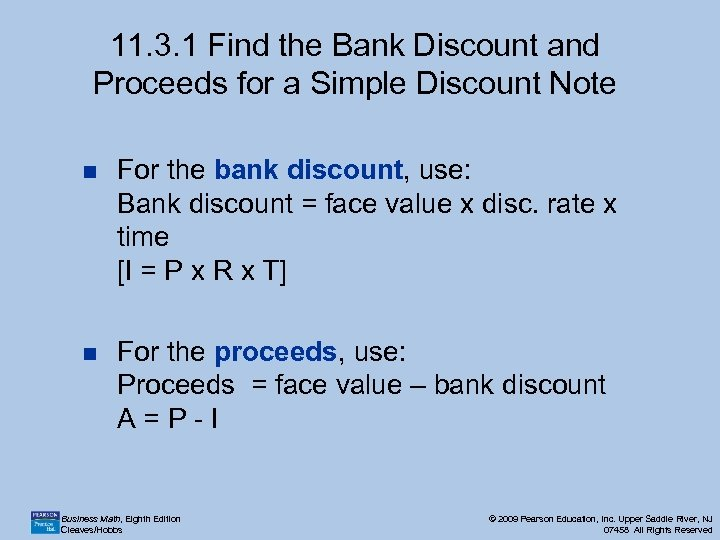11. 3. 1 Find the Bank Discount and Proceeds for a Simple Discount Note n For the bank discount, use: Bank discount = face value x disc. rate x time [I = P x R x T] n For the proceeds, use: Proceeds = face value – bank discount A=P-I Business Math, Eighth Edition Cleaves/Hobbs © 2009 Pearson Education, Inc. Upper Saddle River, NJ 07458 All Rights Reserved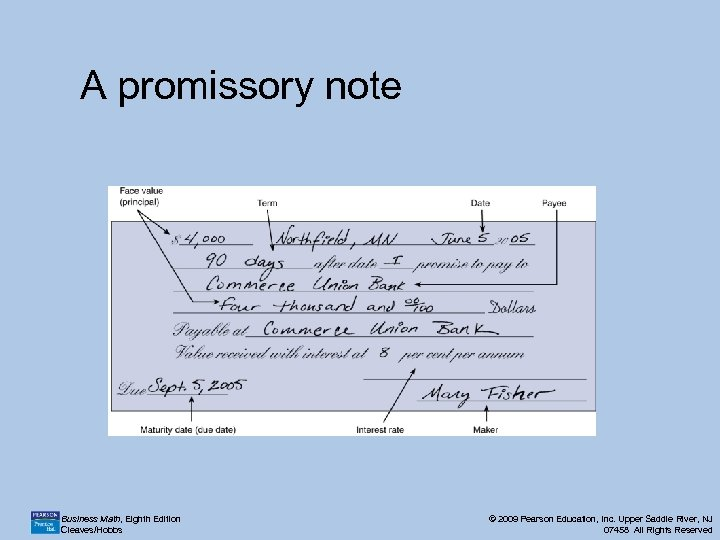A promissory note Business Math, Eighth Edition Cleaves/Hobbs © 2009 Pearson Education, Inc. Upper Saddle River, NJ 07458 All Rights Reserved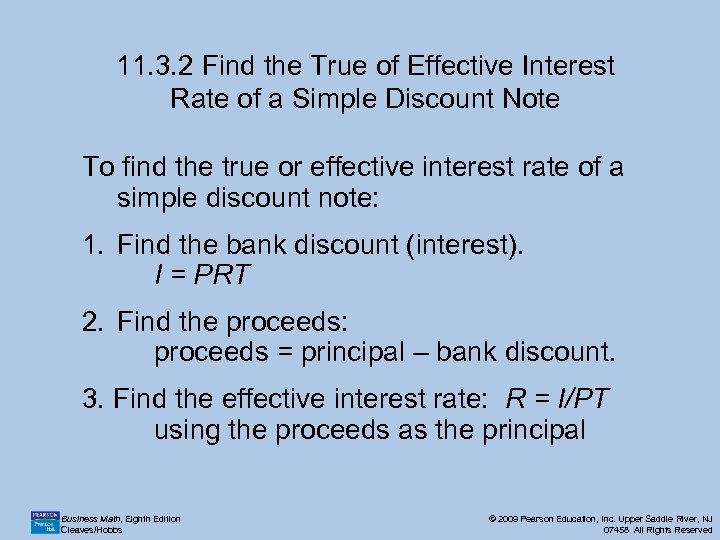11. 3. 2 Find the True of Effective Interest Rate of a Simple Discount Note To find the true or effective interest rate of a simple discount note: 1. Find the bank discount (interest). I = PRT 2. Find the proceeds: proceeds = principal – bank discount. 3. Find the effective interest rate: R = I/PT using the proceeds as the principal Business Math, Eighth Edition Cleaves/Hobbs © 2009 Pearson Education, Inc. Upper Saddle River, NJ 07458 All Rights Reserved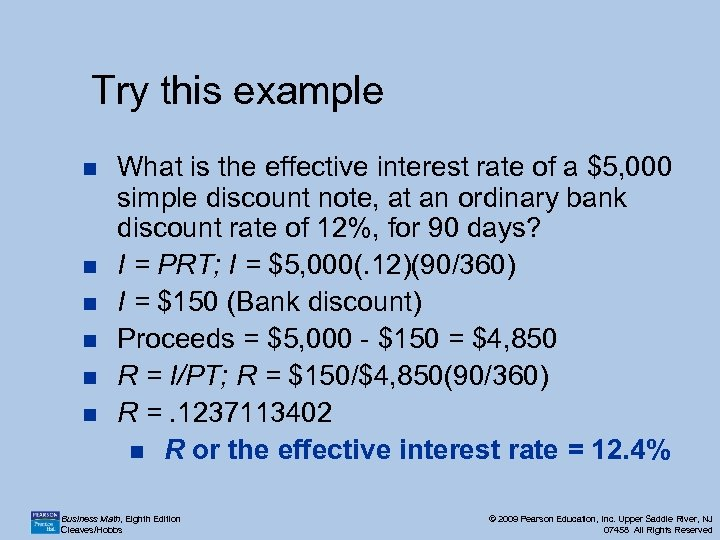Try this example n n n What is the effective interest rate of a \$5, 000 simple discount note, at an ordinary bank discount rate of 12%, for 90 days? I = PRT; I = \$5, 000(. 12)(90/360) I = \$150 (Bank discount) Proceeds = \$5, 000 - \$150 = \$4, 850 R = I/PT; R = \$150/\$4, 850(90/360) R =. 1237113402 n R or the effective interest rate = 12. 4% Business Math, Eighth Edition Cleaves/Hobbs © 2009 Pearson Education, Inc. Upper Saddle River, NJ 07458 All Rights Reserved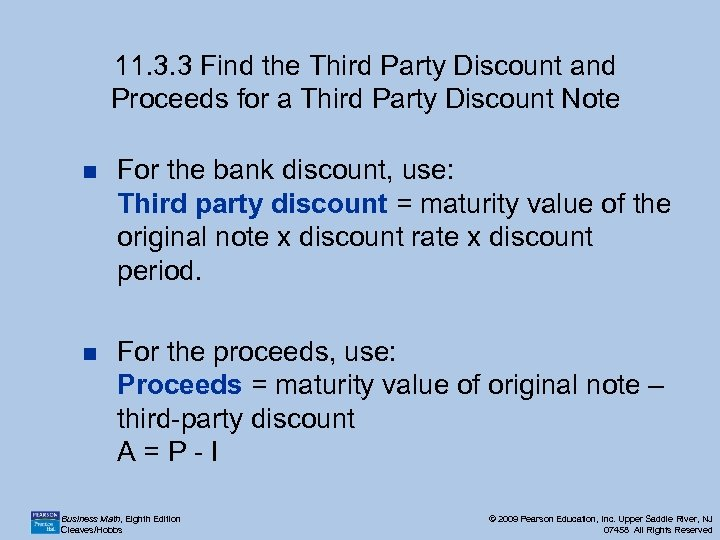11. 3. 3 Find the Third Party Discount and Proceeds for a Third Party Discount Note n For the bank discount, use: Third party discount = maturity value of the original note x discount rate x discount period. n For the proceeds, use: Proceeds = maturity value of original note – third-party discount A=P-I Business Math, Eighth Edition Cleaves/Hobbs © 2009 Pearson Education, Inc. Upper Saddle River, NJ 07458 All Rights Reserved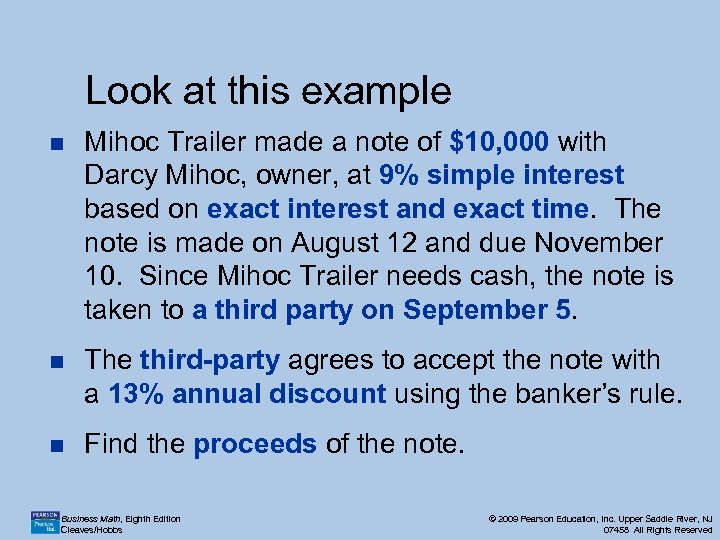Look at this example n Mihoc Trailer made a note of \$10, 000 with Darcy Mihoc, owner, at 9% simple interest based on exact interest and exact time. The note is made on August 12 and due November 10. Since Mihoc Trailer needs cash, the note is taken to a third party on September 5. n The third-party agrees to accept the note with a 13% annual discount using the banker’s rule. n Find the proceeds of the note. Business Math, Eighth Edition Cleaves/Hobbs © 2009 Pearson Education, Inc. Upper Saddle River, NJ 07458 All Rights Reserved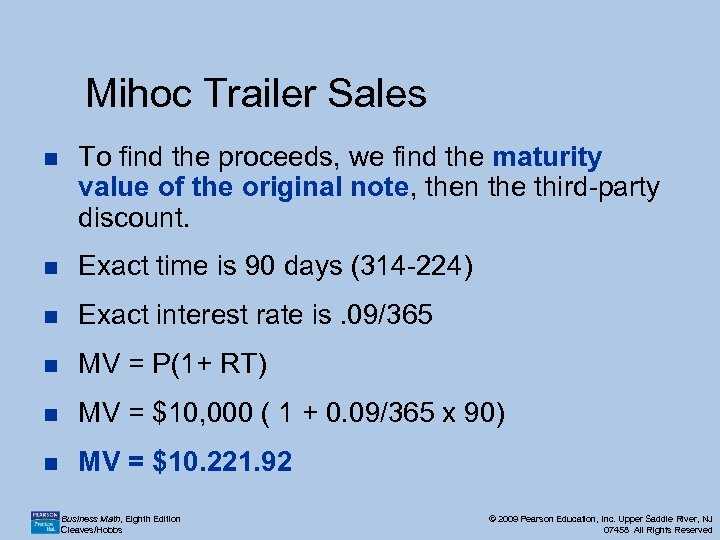Mihoc Trailer Sales n To find the proceeds, we find the maturity value of the original note, then the third-party discount. n Exact time is 90 days (314 -224) n Exact interest rate is. 09/365 n MV = P(1+ RT) n MV = \$10, 000 ( 1 + 0. 09/365 x 90) n MV = \$10. 221. 92 Business Math, Eighth Edition Cleaves/Hobbs © 2009 Pearson Education, Inc. Upper Saddle River, NJ 07458 All Rights Reserved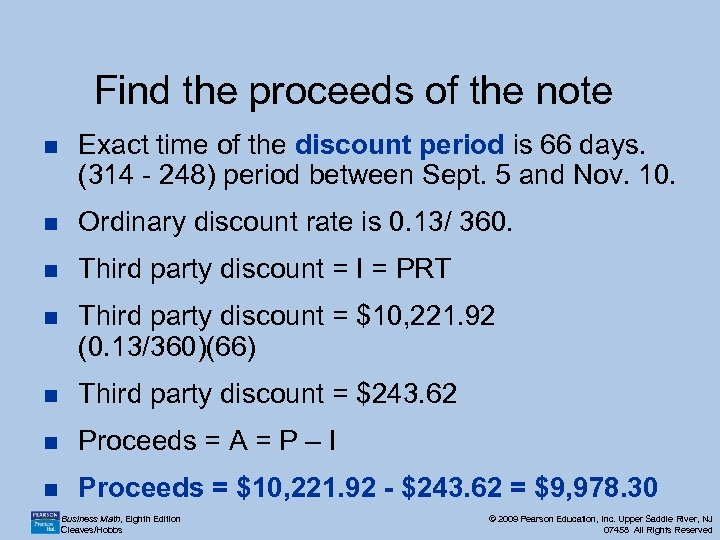Find the proceeds of the note n Exact time of the discount period is 66 days. (314 - 248) period between Sept. 5 and Nov. 10. n Ordinary discount rate is 0. 13/ 360. n Third party discount = I = PRT n Third party discount = \$10, 221. 92 (0. 13/360)(66) n Third party discount = \$243. 62 n Proceeds = A = P – I n Proceeds = \$10, 221. 92 - \$243. 62 = \$9, 978. 30 Business Math, Eighth Edition Cleaves/Hobbs © 2009 Pearson Education, Inc. Upper Saddle River, NJ 07458 All Rights Reserved